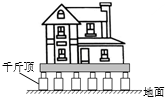2016年3月，南京市将一座民国古建筑整体平移。先用几十个千斤顶将该建筑顶起，如图所示。然后安放在大型平板车上，将该古建筑平移到目的地。古建筑加上防护设施，总质量达到1300t，全部长度达20m。

（1）大型平板车用8min时间将古建筑平移了2400m，则平板车在这段时间内的平均速度是多少？若中间经过一长为80m小巷，则平板车完全通过小巷用多长时间？

（2）若地面能承受的最大压强不能超过5×105Pa，则在顶起该建筑的过程中，所有千斤顶底部与地面的接触面积至少要多大？$\mathrm{v}=\frac{\mathrm{s}}{\mathrm{t}}=\frac{2400 \mathrm{~m}}{8 \times 60 \mathrm{~s}}=5 \mathrm{~m} / \mathrm{s}$,

$\mathrm{t}^{\prime}=\frac{\mathrm{s}^{\prime}}{\mathrm{v}}=\frac{100 \mathrm{~m}}{5 \mathrm{~m} / \mathrm{s}}=20 \mathrm{~s}$;
(2) 古建筑加上防护设施对地面的压力:
$\mathrm{F}=\mathrm{G}=1300 \times 10^3 \mathrm{~kg} \times 10 \mathrm{~N} / \mathrm{kg}=1.3 \times 10^7 \mathrm{~N}$,

$\mathrm{S}=\frac{\mathrm{F}}{\mathrm{p}}=\frac{1.3 \times 10^7 \mathrm{~N}}{5 \times 10^5 \mathrm{~Pa}}=26 \mathrm{~m}^2 。$

（2）若地面能承受的最大压强不能超过 $5 \times 10^5 \mathrm{~Pa}$ ，则在顶起该建筑的过程中，所有干斤顶底部与地面 的接触面积至少要 $26 \mathrm{~m}^2$ 。